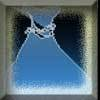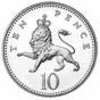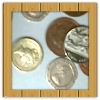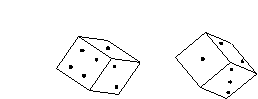#### You may also like### Marbles and Bags

Two bags contain different numbers of red and blue marbles. A marble is removed from one of the bags. The marble is blue. What is the probability that it was removed from bag A?### Coin Tossing Games

You and I play a game involving successive throws of a fair coin. Suppose I pick HH and you pick TH. The coin is thrown repeatedly until we see either two heads in a row (I win) or a tail followed by a head (you win). What is the probability that you win?### Win or Lose?

A gambler bets half the money in his pocket on the toss of a coin, winning an equal amount for a head and losing his money if the result is a tail. After 2n plays he has won exactly n times. Has he more money than he started with?

# Gambling at Monte Carlo

##### Age 14 to 16Challenge LevelAt first you may think that all the probabilities are the same, but consider a similar problem about tossing coins. The probability of one head with one coin is 1/2, the probability of two heads with two coins is 1/4 and the probability of three heads with three coins is 1/8, definitely not the same!

The problem given was difficult and it might be best understood by considering a similar, but simpler, problem.

Which is more likely, at least 1 six from rolling two dice or at least 2 sixes from rolling 4 dice?

To find the solution we use the fact that the probabilities must sum to 1:

The probability of at least 1 six from 2 dice = 1 - probability of no sixes from 2 dice
\begin{align*}
&= 1 - \left(\frac{5}{6}\right)^2 \\
&= 1 - \frac{25}{36} \\
&= \frac{11}{36} \\
\end{align*}

The probability of at least 2 sixes from 4 dice = 1 - probability of no sixes from 4 dice - probability of 1 six from 4 dice
\begin{align*}
&= 1 - \left(\frac{5}{6}\right)^{4} - 12\times\frac{1}{6}\times\left(\frac{5}{6}\right)^{3} \\
&= 1 - \frac{625}{1296} - \frac{500}{1296} \\
& = \frac{171}{1296} \\
\end{align*}

A similar argument for the original problem follows, where $\mathbb{P}(\text{something})$ means 'the probability of something occurring'.

\begin{align*}
\mathbb{P}(\text{at least 1 six from 6 dice}) &= 1 - \left(\frac{5}{6}\right)^6 \\
\end{align*}

\begin{align*}
\mathbb{P}(\text{at least 2 sixes from 12 dice}) &= 1 - \left(\frac{5}{6}\right)^{12} - 12\times\frac{1}{6}\times\left(\frac{5}{6}\right)^{11} \\